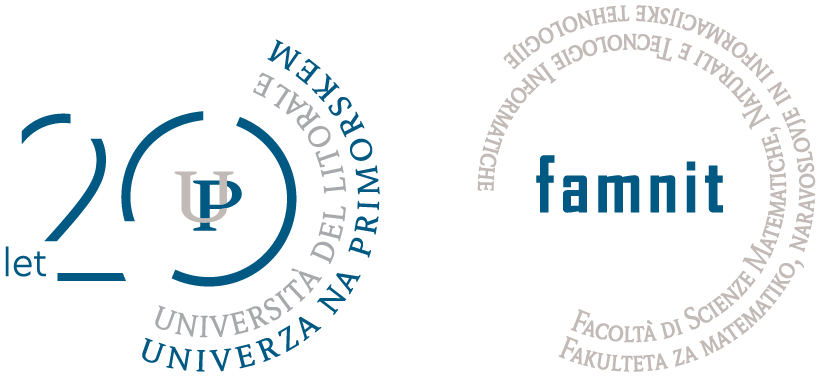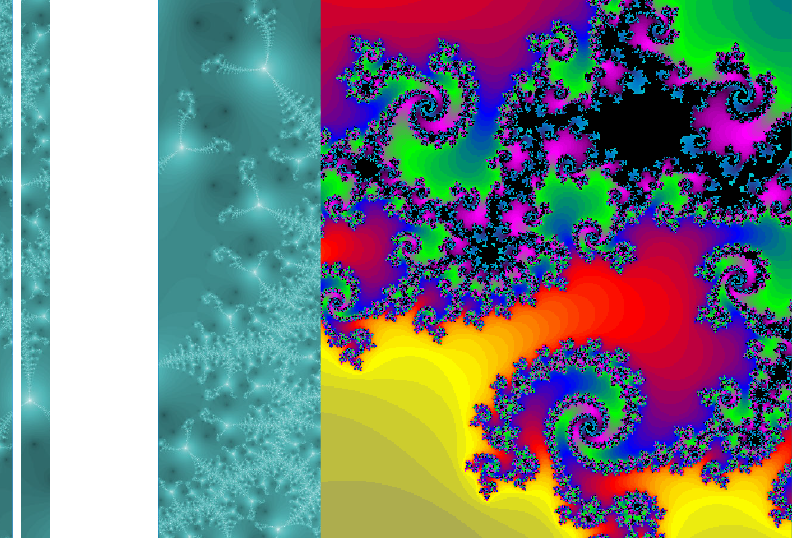Univerza na Primorskem Fakulteta za matematiko, naravoslovje in informacijske tehnologijeSI | EN# Raziskovalni matematični seminar - Arhiv

 2023 2022 2021 2020 2019 2018 2017 2016 2015 2014 2013 2012 2011 2010
 1 2 3 4 5 6 7 8 9 10 11 12
Datum in ura / Date and time: 1.12.14
(10:00-10:45)
Predavalnica / Location: FAMNIT-SEMIN
Predavatelj / Lecturer: dr. Francesco Belardo (UP FAMNIT)
Naslov / Title: Spectral determination of signed graphs
Vsebina / Abstract:

A signed graph is pair (G,s) where G is a graph and s, the signature, is a function on the edges of G assigning values in {1,-1}. Similarly to simple unsigned graphs, it is possible to associate several graph matrices and to study the signed graphs from a spectral viewpoint. A common problem in Spectral Theory of unsigned graphs is to consider special families of graphs and to study whether each graph in the family is determined by the spectrum of the corresponding graph matrix. Namely, a graph is determined by the spectrum if and only if any other cospectral graph is isomorphic as well. In this seminar we extend the latter concept to signed graphs and as an example we will show that the signed Lollipop graph is determined by the spectrum of its Laplacian matrix.

This is a joint research with Pawel Petecki.

Datum in ura / Date and time: 24.11.14
(10:00-10:45)
Predavalnica / Location: FAMNIT-SEMIN
Predavatelj / Lecturer: Dr. Jurij Kovič
Naslov / Title: Japonska tempeljska geometrija

Datum in ura / Date and time: 17.11.14
(10:00-10:45)
Predavalnica / Location: FAMNIT-SEMIN
Predavatelj / Lecturer: Nina Chiarelli
Naslov / Title: Equistarable bipartite graphs
Vsebina / Abstract:

Recently, Milanič and Trotignon introduced the class of equistarable graphs as graphs without isolated vertices admitting positive vertex weights on the edges such that a subset of edges is of total weight 1 if and only if it forms a maximal star. Based on equistarable graphs, counterexamples to three conjectures on equistable graphs were constructed, in particular to Orlin's conjecture, which states that every equistable graph is a general partition graph.

In the talk we characterize equistarable bipartite graphs.

We show that a bipartite graph is equistarable if and only if every 2-matching of the graph extends to a matching covering all vertices of degree at least 2. As a consequence of this result, we obtain that Orlin's conjecture holds within the class of complements of line graphs of bipartite graphs.

We also connect equistarable graphs to the triangle condition, a combinatorial condition known to be necessary (but in general not  sufficient) for equistability. We show that the triangle condition implies general partitionability for complements of line graphs of forests, and construct an infinite family of triangle non-equistable graphs within the class of complements of line graphs of bipartite graphs.

This is a joint work with Martin Milanič and Endre Boros.

Datum in ura / Date and time: 10.11.14
(10:00-10:45)
Predavalnica / Location: FAMNIT-SEMIN
Predavatelj / Lecturer: prof. dr. Tomaž Pisanski
Naslov / Title: General Haar graphs in sage
Vsebina / Abstract:

We implemented several programs that may deal with general Haar graphs. The programs are written in Python but embedded in the system sage. A Haar graph is a covering graph over a dipole. This means it can be described by a set
S of voltages where each element s from S is assigned to one of the one of the arcs leading from a black vertex of a dipole to a white vertex of a dipole with k edges, such that |S| = k and each arc receives its voltage.  Here S is a set taken from some group G. If X is a set on n vertices, then each faithful X-action of G gives rise to a derived graph H(S,G,X), called the Haar graph. In paractice only two actions are considered:
1. G is a subgroup of the symmetric group Sym(X), generated by S. In this case S is simply a set of permutations of an n-set. We shorten notation to H(S) and call the derived graph a permutation Haar graph.
2. G is generated by S and acts on itself with the usual right regular action. We shorten the notation to H(S,G) and call the derived graph a G-Haar graph. In particular, the cyclic Haar graphs have been studied in the past by Hladnik, Marusic and Pisanski. Hladnik independently developed a theory that lead to the study of abelian Haar graphs.

Our system has possibility to work with special classes of Haar graphs, eg. permutation Haar graph, cyclic Haar graph and abelian Haar graphs.

Datum in ura / Date and time: 3.11.14
(10:00-10:45)
Predavalnica / Location: FAMNIT-SEMIN
Predavatelj / Lecturer: dr. Rok Požar
Naslov / Title: Solvable regular covering projections of graphs
Vsebina / Abstract:

We present basic properties of universal covering projections and exploit them in order to develop an algorithm for computing all solvable regular covering projections of a given graph admitting a lift of a given group of automorphisms.